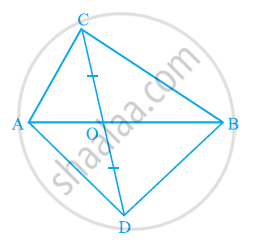# In the given figure, ABC and ABD are two triangles on the same base AB. If line-segment CD is bisected by AB at O, show that ar (ABC) = ar (ABD). - Mathematics

In the given figure, ABC and ABD are two triangles on the same base AB. If line-segment CD is bisected by AB at O, show that ar (ABC) = ar (ABD).#### Solution

Consider ΔACD.

Line-segment CD is bisected by AB at O. Therefore, AO is the median of

ΔACD.

∴ Area (ΔACO) = Area (ΔADO) ... (1)

Considering ΔBCD, BO is the median.

∴ Area (ΔBCO) = Area (ΔBDO) ... (2)

Adding equations (1) and (2), we obtain

Area (ΔACO) + Area (ΔBCO) = Area (ΔADO) + Area (ΔBDO)

⇒ Area (ΔABC) = Area (ΔABD)

Concept: Corollary: Triangles on the same base and between the same parallels are equal in area.
Is there an error in this question or solution?
Chapter 9: Areas of Parallelograms and Triangles - Exercise 9.3 [Page 162]

#### APPEARS IN

NCERT Class 9 Maths
Chapter 9 Areas of Parallelograms and Triangles
Exercise 9.3 | Q 4 | Page 162

Share